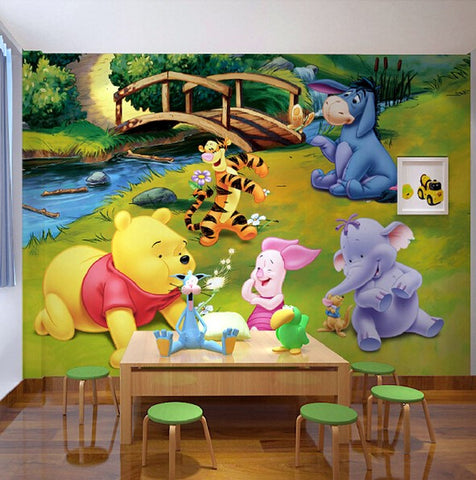## Winnie the Pooh Tiger Cartoon Wall Mural Stereoscopic Wallpaper

• \$39.95

Product Details
• Style: For Kids
• Function: Moisture-Proof, Mold-Proof, Smoke-Proof, Heat Insulation, Anti-static
• Features: Formaldehyde-free, Mildew Resistant, Extra Thick, Environment Friendly
• Surface Treatment: Embossed
• Material: Plant, Non-Woven
• Pattern: Winnie the Pooh Cartoon
• Can be Customized?: Yes

Materials: Straw Texture (default material)/ Leather Pattern/ 3D Relief/ Waterproof Polyester oil canvas.  Straw texture is the default material we ship.  If you prefer one of the others, please let us know when you order.

Important Please Read! \$39.95 is for 1 piece. only.  It is very small, about 4'7" x 2'4". To cover a complete wall you will need more pieces.  The example pictures show a wall that is fully covered with wallpaper.  You need to measure your wall and calculate how many pieces you need to order.  When you know, go to checkout and select the number you wish to purchase. Total will be calculated for you.

How to calculate how many pieces you need:
1). Measure your wall.  Convert your measurements to centimeters (you can use any online metric converter). 2). If your wall is 360cm width x 240cm height for example,  multiply those numbers: 360 x 240 = 8.6.  So you would need to buy 9 pieces.

Here are some fixed sizes:

Quantity 1  :  1 square meter = 140cm(W) x 70cm(H) (4'7" x 2'4")

Quantity 2  :  2 square meter = 200cm(W) x 100cm(H) (6'7" x 3'3")

Quantity 3  :  3 square meter = 220cm(W) x 140cm(H) (7'3" x 4'7")

Quantity 4  :  4 square meter = 250cm(W) x 160cm(H) (8'2" x 5'3")

Quantity 5  :  5 square meter = 280cm(W) x 180cm(H) (9'2" x 5'11")

Quantity 6  :  6 square meter = 300cm(W) x 200cm(H) (9'10" x 6'7")

Quantity 7  :  7 square meter = 330cm(W) x 210cm(H) (10'10" x 6'11")

Quantity 8  :  8 square meter = 360cm(W) x 230cm(H) (11'10" x 7'6")

Quantity 9  :  9 square meter = 380cm(W) x 240cm(H) (12'5" x 7'10")

Quantity 10 :  10 square meter = 400cm(W) x 250cm(H) (13'1" x 8'2")

Quantity 11 : 11 square meter = 420cm(W) x 260cm(H) (13'9'' x 8'6'')

Quantity 12 : 12 square meter = 440cm(W) x 270cm(H) (14'5" x 8'10")

Quantity 13 : 13 square meter = 460cm(W) x 280cm(H) (15'1'' x 9'2'')

Quantity 14 : 14 square meter = 480cm(W) x 290cm(H) (15'9'' x 9'6'')

Quantity 15 : 15 square meter= 500cm(W) x 300cm(H) (16'5'' x 9'10'')

Quantity 16 : 16 square meter=500cm(W) x 320cm(H) (16'5" x 10'6")

We Also Recommend